Related Articles

# Split Pandas Dataframe by Column Index

• Difficulty Level : Hard
• Last Updated : 29 Aug, 2020

Pandas support two data structures for storing data the series (single column) and dataframe where values are stored in a 2D table (rows and columns).  To index  a  dataframe using the index we need to make use of dataframe.iloc() method which takes

Syntax: pandas.DataFrame.iloc[]

Parameters:
Index Position: Index position of rows in integer or list of integer.

Return type: Data frame or Series depending on parameters

Let’s create a dataframe. In the below example we will use a simple binary dataset used to classify if a species is a mammal or reptile. The species column holds the labels where 1 stands for mammal and 0 for reptile. The data is stored in the dict which can be passed to the DataFrame function outputting a dataframe.

## Python3

 `import` `pandas as pd`` ` `dataset ``=` `{``'toothed'``: [``1``, ``1``, ``1``, ``0``, ``1``, ``1``, ``1``, ``1``, ``1``, ``0``],``           ``'hair'``: [``1``, ``1``, ``0``, ``1``, ``1``, ``1``, ``0``, ``0``, ``1``, ``0``],``           ``'breathes'``: [``1``, ``1``, ``1``, ``1``, ``1``, ``1``, ``0``, ``1``, ``1``, ``1``],``           ``'legs'``: [``1``, ``1``, ``0``, ``1``, ``1``, ``1``, ``0``, ``0``, ``1``, ``1``],``           ``'species'``: [``1``, ``1``, ``0``, ``1``, ``1``, ``1``, ``0``, ``0``, ``1``, ``0``]``           ``}`` ` `df ``=` `pd.DataFrame(dataset)`` ` `df.head()`

Output :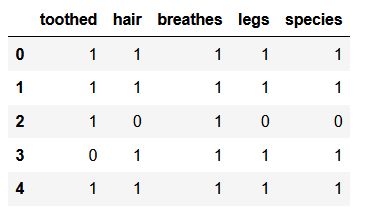Example 1: Now we would like to separate species columns from the feature columns (toothed, hair, breathes, legs) for this we are going to make use of the iloc[rows, columns] method offered by pandas.

Here ‘:’ stands for all the rows and -1 stands for the last column so the below cell is going to take the all the rows and all columns except the last one (‘species’) as can be seen in the output:

## Python3

 `X ``=` `df.iloc[:,:``-``1``]``X`

Output: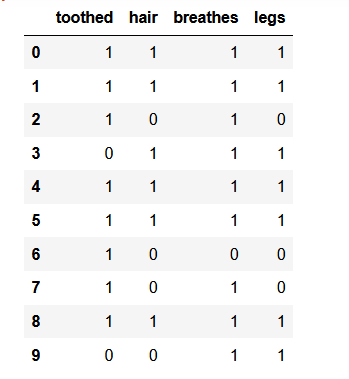To split the species column from the rest of the dataset we make you of a similar code except in the cols position instead of padding a slice we pass in an integer value -1.

## Python3

 `Y ``=` `df.iloc[:,``-``1``]``Y`

Output :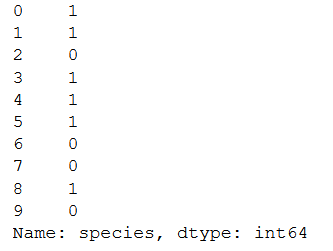Example 2: Splitting using list of integers

Similar output can be obtained by passing in a list of integers instead of a slice

## Python3

 `X ``=` `df.iloc[:,[``0``,``1``,``2``,``3``]]``X`

Output:To the species column we are going to use the index of the column which is 4 we can use -1 as well

## Python3

 `Y ``=` `df.iloc[:,``4``]``Y`

Output:Example 3: Splitting dataframes into 2 separate dataframes

In the above two examples, the output for Y was a Series and not a dataframe Now we are going to split the dataframe into two separate dataframe’s this can be useful when dealing with multi-label datasets. Will be using the same dataset.

In the first, we are going to split at column hair

## Python3

 `df.iloc[:,[``0``,``1``]]`

Output: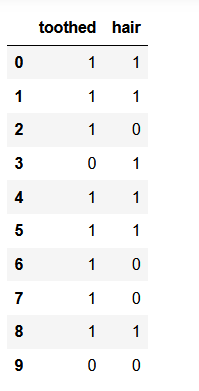The second dataframe will contain 3 columns breathes , legs , species

## Python3

 `df.iloc[:,[``2``,``3``,``4``]] `

Output: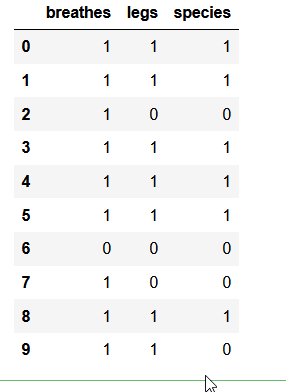Attention geek! Strengthen your foundations with the Python Programming Foundation Course and learn the basics.

To begin with, your interview preparations Enhance your Data Structures concepts with the Python DS Course. And to begin with your Machine Learning Journey, join the Machine Learning – Basic Level Course

My Personal Notes arrow_drop_up